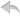注册    登录创作新主题

Python
 python开源   Django   Python   DjangoApp   pycharm
DATA
 docker   Elasticsearch

 分享发现   分享创造   问与答   求职   闲聊   区块链   招聘   支付之战   翻译   创业
WEB开发
 linux   bottle   tornado   DATABASE   IE   NoSql   NGINX   MQ   Bootstrap   js   其他Web框架   Jquery   peewee   web工具   MongoDB   Git   zookeeper   Redis

Python88.com
 社区推广   反馈   公告
Python社区  »  Python

# python:附加记录不会附加到文件

WhiteGlint • 1 周前 • 7 次点击

``````run_again = 'y'

while run_again == 'y':
widget = 10.55
gidget = 7.30
print('1 - Create a new Invoice Order')
print('2 - Add an additional Invoice Order')
print('3 - Display all Invoice Orders')
print('4 - QUIT')
choice = int(input('Enter 1,2,3, or 4: '))
if (choice == 1):
invoice_file = open('invoices.txt', 'w')
ID = input('1 of 4 - Enter customer ID: ')
numwidgets = int(input('2 of 4 - Enter number of Widgets'
' Ordered @ \$10.55 each: '))
numgidgets = int(input('3 of 4 - Enter number of Gidgets'
'(Ordered @ \$7.50 each): '))
numdoodads = int(input('4 of 4 - Enter number of Doodads'
'(Ordered @ \$5.25 each: '))
invoice_file.write(ID + '\n')
invoice_file.write(str(numwidgets) + '\n')
invoice_file.write(str(numgidgets) + '\n')

invoice_file.close()
print('Data has been added to file invoices.txt')

if (choice == 2):
invoice_file = open('invoices.txt', 'a')
ID = input('1 of 4 - Enter customer ID: ')
numwidgets = int(input('2 of 4 - Enter number of Widgets'
' Ordered @ \$10.55 each: '))
numgidgets = int(input('3 of 4 - Enter number of Gidgets'
'(Ordered @ \$7.50 each): '))
numdoodads = int(input('4 of 4 - Enter number of Doodads'
'(Ordered @ \$5.25 each: '))
invoice_file.write(ID + '\n')
invoice_file.write(str(numwidgets) + '\n')
invoice_file.write(str(numgidgets) + '\n')

invoice_file.close()
print('Data has been added to file invoices.txt')
if (choice == 3):
invoice_file = open('invoices.txt', 'r')
records = 0
records += 1
widgettotal = widget * numwidgets
gidgettotal = gidget * numgidgets
invoicetotal = widgettotal + gidgettotal + doodadtotal

print('Record #',records, 'Customer #',ID)
print(numwidgets, 'Widgets ordered @ \$10.55---- \$',widgettotal)
print(numgidgets, 'Gidgets ordered @ \$7.30----\$',gidgettotal)
print('Invoice total------------\$',invoicetotal)

invoice_file.close()

if (choice == 4):
break

run_again = input('Would you like to run this program again?'
'(Enter y for yes or n for no): ')
``````
Python社区是高质量的Python/Django开发社区•   1 楼 Quinn Mortimer    9 月前 我认为问题不一定是选项2中的行没有写入文件,而是它们没有作为选项3的一部分被读取。选项3中的代码将尝试读取文件的前四行,而不读取其他任何内容。相反,你可能想要这样的东西: ``````if choice == "3": invoice_file = open('invoices.txt', 'r') lines = invoice_file.readlines(4) records, num_widgets, num_gadgets, num_doodads = 0, 0, 0, 0 while len(lines) == 4: records += 1 num_widgets += 1 # ... lines = invoice_file.readlines(4) # Do calculations, output, and close file ``````